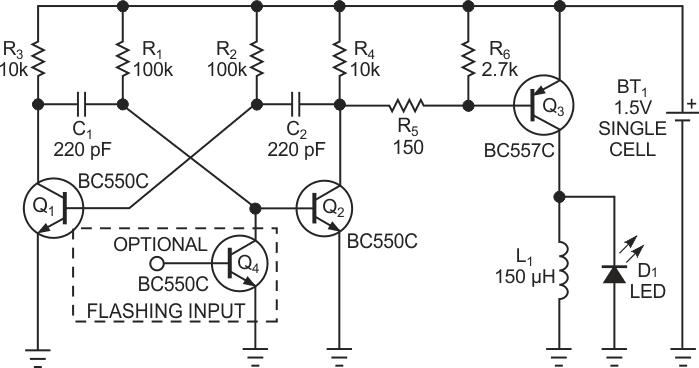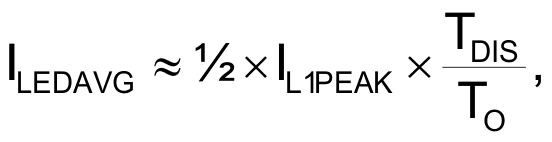# Astable multivibrator lights LED from a single cell

## Fairchild BC550C

Lighting LEDs from a single 1.5 V cell poses a problem because their forward voltages are higher than the cell’s. The simplest way to light the LED is to use a step-up dc/dc converter. This Design Idea offers a simple and reliable alternative for applications in which low cost is of primary concern. The circuit in Figure 1 employs a classic astable oscillator, which transistors Q1 and Q2 form. The square-wave drive signal at Q2’s collector turns a PNP switching transistor, Q3, on and off. When Q3 turns on, it charges inductor L1, and, when it turns off, inductor L1 discharges its stored energy through the LED during flyback, allowing you to light any type or color of LED.Figure 1. This simple astable multivibrator provides a low-cost way to drive an LED from a single cell.

The astable circuit oscillates at a frequency of 1/TO, where TO = TL + TH with TL ≈ 0.76R2•C2 and TH ≈ 0.76R1•C1 when the cell voltage is 1.5 V, where TO is the time, TL is the on-time, and TH is the off-time. With the component values in Figure 1, the frequency and the duty cycle are about 28.5 kHz and 50%, respectively. During the on-time, transistor Q3 is on, and inductor L1 starts to charge with constant voltage so its current ramps up linearly to a peak value, as the following equation describes:where IL1PEAK is the peak current of L1, VBAT is the battery voltage, and VCESATQ3 is the collector-to-emitter saturation voltage of Q3. During the off-time, Q3 is off, and the inductor’s voltage reverses polarity, forward-biasing the LED and discharging through it at a constant voltage roughly equal to the forward voltage of the LED while its current ramps down to zero.

Because this cycle repeats at a high rate, the LED appears always on. The LED’s brightness depends on its own average current, which is proportional to the peak value. Because the LED current is roughly a triangular pulse with a peak current approximately equal to the inductor’s current because of the finite turn-off time of Q3, you can easily estimate the average current:where TDIS is the discharge time of inductor L1 through the LED, which you can roughly estimate from the slope of L1’s discharge, which is VLED/L1, where VLED is the LED’s voltage.

To control the LED’s brightness, you may increase or decrease the inductor’s peak current by varying its inductance from 100 to 330 µH to achieve the optimal brightness for the type of LED you are using. However, L1’s charge slope is always smaller than its discharge slope, and, because TL and TH are equal, L1 has enough time to discharge completely. When it recharges on its next cycle, its current cycle always starts from zero. If this is not the case – if you reduce TH too much, for example – the inductor current increases on each cycle until Q3 goes out of saturation, and the final current value becomes unpredictable because it depends on Q3’s dc gain. Optional transistor Q4 allows the circuit to flash the LED when a low-frequency gating signal drives its base.

No one component is critical; for example, any small-signal transistor is suitable. But, if possible, choose a PNP transistor for Q3 with high dc-current gain and low collector-to-emitter saturation voltage for best efficiency. Also, take care that the peak current does not saturate L1 and does not exceed the maximum peak-current rating of Q3 and the LED. The astable circuit starts to operate with a supply voltage as low as 0.6 V, but the LED is off and begins to light dimly when the supply voltage exceeds 0.9 V. When the supply voltage exceeds 1 V, the LED’s brightness is adequate, even if it depends slightly on the forward voltage of the LED.

EDN